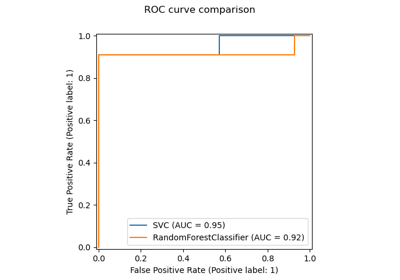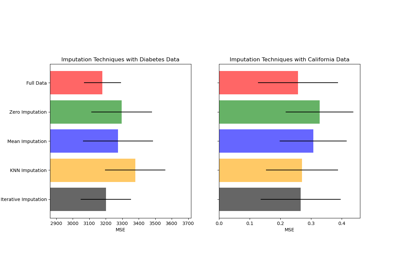# sklearn.impute.KNNImputer¶

class sklearn.impute.KNNImputer(*, missing_values=nan, n_neighbors=5, weights='uniform', metric='nan_euclidean', copy=True, add_indicator=False, keep_empty_features=False)[source]

Imputation for completing missing values using k-Nearest Neighbors.

Each sample’s missing values are imputed using the mean value from n_neighbors nearest neighbors found in the training set. Two samples are close if the features that neither is missing are close.

Read more in the User Guide.

New in version 0.22.

Parameters:
missing_valuesint, float, str, np.nan or None, default=np.nan

The placeholder for the missing values. All occurrences of missing_values will be imputed. For pandas’ dataframes with nullable integer dtypes with missing values, missing_values should be set to np.nan, since pd.NA will be converted to np.nan.

n_neighborsint, default=5

Number of neighboring samples to use for imputation.

weights{‘uniform’, ‘distance’} or callable, default=’uniform’

Weight function used in prediction. Possible values:

• ‘uniform’ : uniform weights. All points in each neighborhood are weighted equally.

• ‘distance’ : weight points by the inverse of their distance. in this case, closer neighbors of a query point will have a greater influence than neighbors which are further away.

• callable : a user-defined function which accepts an array of distances, and returns an array of the same shape containing the weights.

metric{‘nan_euclidean’} or callable, default=’nan_euclidean’

Distance metric for searching neighbors. Possible values:

• ‘nan_euclidean’

• callable : a user-defined function which conforms to the definition of _pairwise_callable(X, Y, metric, **kwds). The function accepts two arrays, X and Y, and a missing_values keyword in kwds and returns a scalar distance value.

copybool, default=True

If True, a copy of X will be created. If False, imputation will be done in-place whenever possible.

If True, a MissingIndicator transform will stack onto the output of the imputer’s transform. This allows a predictive estimator to account for missingness despite imputation. If a feature has no missing values at fit/train time, the feature won’t appear on the missing indicator even if there are missing values at transform/test time.

keep_empty_featuresbool, default=False

If True, features that consist exclusively of missing values when fit is called are returned in results when transform is called. The imputed value is always 0.

New in version 1.2.

Attributes:
indicator_MissingIndicator

Indicator used to add binary indicators for missing values. None if add_indicator is False.

n_features_in_int

Number of features seen during fit.

New in version 0.24.

feature_names_in_ndarray of shape (n_features_in_,)

Names of features seen during fit. Defined only when X has feature names that are all strings.

New in version 1.0.

SimpleImputer

Univariate imputer for completing missing values with simple strategies.

IterativeImputer

Multivariate imputer that estimates values to impute for each feature with missing values from all the others.

References

• Olga Troyanskaya, Michael Cantor, Gavin Sherlock, Pat Brown, Trevor Hastie, Robert Tibshirani, David Botstein and Russ B. Altman, Missing value estimation methods for DNA microarrays, BIOINFORMATICS Vol. 17 no. 6, 2001 Pages 520-525.

Examples

>>> import numpy as np
>>> from sklearn.impute import KNNImputer
>>> X = [[1, 2, np.nan], [3, 4, 3], [np.nan, 6, 5], [8, 8, 7]]
>>> imputer = KNNImputer(n_neighbors=2)
>>> imputer.fit_transform(X)
array([[1. , 2. , 4. ],
[3. , 4. , 3. ],
[5.5, 6. , 5. ],
[8. , 8. , 7. ]])


Methods

 fit(X[, y]) Fit the imputer on X. fit_transform(X[, y]) Fit to data, then transform it. get_feature_names_out([input_features]) Get output feature names for transformation. get_params([deep]) Get parameters for this estimator. set_output(*[, transform]) Set output container. set_params(**params) Set the parameters of this estimator. Impute all missing values in X.
fit(X, y=None)[source]

Fit the imputer on X.

Parameters:
Xarray-like shape of (n_samples, n_features)

Input data, where n_samples is the number of samples and n_features is the number of features.

yIgnored

Not used, present here for API consistency by convention.

Returns:
selfobject

The fitted KNNImputer class instance.

fit_transform(X, y=None, **fit_params)[source]

Fit to data, then transform it.

Fits transformer to X and y with optional parameters fit_params and returns a transformed version of X.

Parameters:
Xarray-like of shape (n_samples, n_features)

Input samples.

yarray-like of shape (n_samples,) or (n_samples, n_outputs), default=None

Target values (None for unsupervised transformations).

**fit_paramsdict

Returns:
X_newndarray array of shape (n_samples, n_features_new)

Transformed array.

get_feature_names_out(input_features=None)[source]

Get output feature names for transformation.

Parameters:
input_featuresarray-like of str or None, default=None

Input features.

• If input_features is None, then feature_names_in_ is used as feature names in. If feature_names_in_ is not defined, then the following input feature names are generated: ["x0", "x1", ..., "x(n_features_in_ - 1)"].

• If input_features is an array-like, then input_features must match feature_names_in_ if feature_names_in_ is defined.

Returns:
feature_names_outndarray of str objects

Transformed feature names.

get_params(deep=True)[source]

Get parameters for this estimator.

Parameters:
deepbool, default=True

If True, will return the parameters for this estimator and contained subobjects that are estimators.

Returns:
paramsdict

Parameter names mapped to their values.

set_output(*, transform=None)[source]

Set output container.

See Introducing the set_output API for an example on how to use the API.

Parameters:
transform{“default”, “pandas”}, default=None

Configure output of transform and fit_transform.

• "default": Default output format of a transformer

• "pandas": DataFrame output

• None: Transform configuration is unchanged

Returns:
selfestimator instance

Estimator instance.

set_params(**params)[source]

Set the parameters of this estimator.

The method works on simple estimators as well as on nested objects (such as Pipeline). The latter have parameters of the form <component>__<parameter> so that it’s possible to update each component of a nested object.

Parameters:
**paramsdict

Estimator parameters.

Returns:
selfestimator instance

Estimator instance.

transform(X)[source]

Impute all missing values in X.

Parameters:
Xarray-like of shape (n_samples, n_features)

The input data to complete.

Returns:
Xarray-like of shape (n_samples, n_output_features)

The imputed dataset. n_output_features is the number of features that is not always missing during fit.

## Examples using sklearn.impute.KNNImputer¶Release Highlights for scikit-learn 0.22

Release Highlights for scikit-learn 0.22Imputing missing values before building an estimator

Imputing missing values before building an estimator